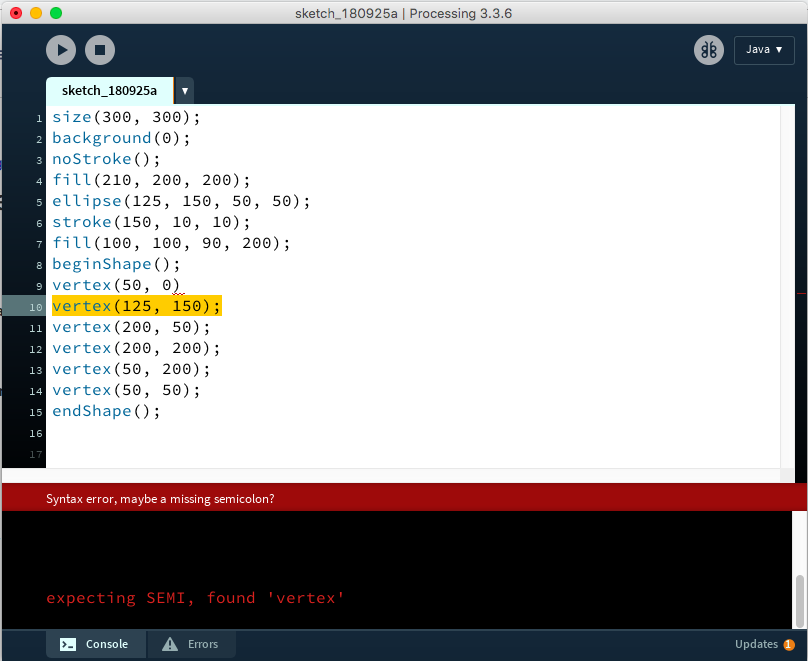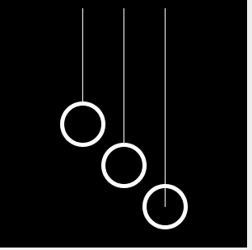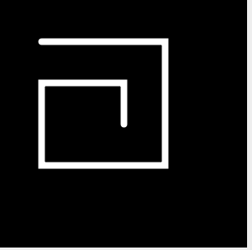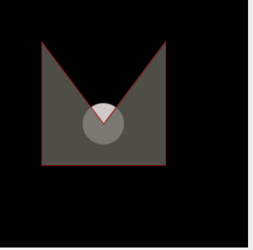# Starting with Processing (en)

### Installing Processing

Processing is a programming environment (Integrated development environment IDE) based on the programming language Java. Processing is Open Source and you can download it for free here. Solutions to common installation problems can be found here

The Processing IDE (Integrated development environment)

The Processing IDE interface consists of three main parts. First is the text editor, this is where we can write (or paste in) our code. Then there is the Message area and the Console/error window. The Message error will show us some hints if we do something wrong, like forget to put a semicolon at the end of every line. The Console/Error window is similar in that it can display warnings, but we can also use it to output our own text for debugging as we will see in later tutorials. The final part is the Display window. This is where we see the results of our code after it is compiled, by pressing the Run button in the upper left corner.Example 1 - Graphic output

This example shows how to draw graphics directly to an output window. You can find further drawing functions Processing reference page ( link ).```size(300,300); // define window size
background(0); // define background colour
stroke(255,255,255); // define line colour
line(100,10,100,150); // draw a line
line(150,10,150,200);
line(200,10,200,250);
fill(0,0,0); // define fill colour
strokeWeight(5); // line thickness
ellipse(100,150,50,50); // draw an ellipse
ellipse(150,200,50,50); // draw an  ellipse
noFill(); // turn of fill
ellipse(200,250,50,50); // draw an  ellipse```

Example 2 - Complex Forms

This example shows you how to change line attributes and how to draw more complex shapes.```size(300,300); // define window size
background(0); // define background colour
stroke(255); // define line colour
strokeWeight(8); // line thickness
strokeJoin(MITER); // this is the default
//strokeJoin(BEVEL);
//strokeJoin(ROUND);
noFill();
beginShape();
vertex(50,50);
vertex(200,50);
vertex(200,200);
vertex(50,200);
vertex(50,100);
vertex(150,100);
vertex(150,150);
endShape();```

Example 3 colors and transparency

Colours are defined with 3 values, the so-called RGB values. The colour consists of three components (red + green + blue) and is determined by the additive color mixing. The value of a colour component can range from 0-255.

This range of numbers results from the fact that each color value represents an 8-bit value. Therefore, we also speak of true color with 24-bit (3 * 8-bit = RGB).

Forms can also be transparent, using the alpha channel. The colour is simply extended by a 4th parameter (RGBA). Smaller alpha value are more transparent than greater ones.```size(300,300);
background(0);
noStroke();
fill(210,200,200);
ellipse(125,150,50,50);
stroke(150,10,10);
fill(100,100,90,200);
beginShape();
vertex(50,50);
vertex(125,150);
vertex(200,50);
vertex(200,200);
vertex(50,200);
vertex(50,50);
endShape();```

Exercise 1:

Expand and modify the examples with new or combined drawing commands (Arc, Point, Triangle, etc.).